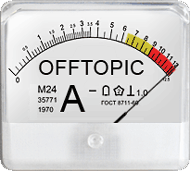## OT: R limbo 101 [Software]Dear Ohlbe,

» I thought something like if... else... would be very logical and easy. Maybe because these are real words from a language I can understand.

Perfectly understandable. Of course, these constructs exist in R and we use them all the time. The point is only that direct access of values (with a condition) is simply more efficient.
If you don’t have to deal with large data sets, both are fine. Since I deal a lot with simulations (1 mio BE studies with a sometimes high number of subjects / periods / sequences, …) I learned the hard way to avoid the ‘simple’ constructs if ever possible (given, sometimes I know that it could be done but was too stupid).
Think about my previous example. For 1 mio calls the direct access is 15times faster than the loop and if() within. It’s not unusual to have nested calls. For two it would by ~230times slower and for three already ~3,500times. Would test your patience.

» But it looks like R and I are following different kinds of logics.

Not necessarily so. See above.

» When you learn a new language and start speaking it with somebody, that person will usually show some goodwill, ignore grammatical mistakes and try and understand what you mean. R made no efforts of any kind, even though what I was trying to achieve was pretty obviousI agree. See the subject line. The man-pages of’s functions were written by statisticians, regularly forgetting non-expert users. Sometimes the response you get is bizarre. Want to know how for() works?

?for + 
+
Fuck, get me out of here!
help(for) Error: unexpected ')' in "help(for)"
What the heck? Shall I really try it without the closing bracket? Strange. OK, OK.
help(for +
Aha, the closing bracket is missing!
)
Error: unexpected ')' in: " )"
?for help(for)
Both work as expected. I don’t know what’s the logic behind. Beyond me.

» » If you have a vector of data (say y)
»
» Yeah, ElMaestro started using this kind of vocabulary too when we were exchanging email. […] All I learnt at school about vectors is the geometric side of the concept - what Wikipedia apparently calls Euclidian vector.

Correct.

» I had some problems understanding what an arrow between two points had to do with what I was trying to achieve.

Absolutely nothing, of course. Ina one-dimensional array is meant. The indexing (i.e., where it starts) differs between languages.
• 0
C, C++, BASIC-dialects, Pascal (default; can be declared as 1), PHP, Perl, Java, JavaScript, Lisp, Python, Ruby, Scheme, …
• 1
S, R, Julia, FORTRAN, ALGOL, AWK, COBOL, Matlab, Mathematica, Wolfram Language, XQuery, …
In many languages there are three types of data structures: scalar (a single number, character, string, boolean), one- (R: vector) and multidimensional array (R: data.frame and matrix). In R there are no scalars, only vectors containing a single element instead.

x1   <- 1 x2   <- 1L x3   <- TRUE x4   <- NA x5   <- "a" comp <- matrix(data = c(c(x1, x2, x3, x4, x5),                         c(is.vector(x1), is.vector(x2), is.vector(x3),                           is.vector(x4), is.vector(x5)),                         c(length(x1), length(x2), length(x3),                           length(x4), length(x5)),                         c(typeof(x1), typeof(x2), typeof(x3),                           typeof(x4),typeof(x5))),                nrow = 5, ncol = 4,                dimnames = list(paste0("x", 1:5),                                c("value", "vector?", "length", "type"))) print(as.data.frame(comp))    value vector? length      type x1     1    TRUE      1    double x2     1    TRUE      1   integer x3  TRUE    TRUE      1   logical x4  <NA>    TRUE      1   logical x5     a    TRUE      1 character

What makesso powerful is yet another data type, namely the list. A list can contain any of the other types and a mixture of them (even other lists…).

char.vect <- letters[1:2] int.vect  <- as.integer(1:3) mydf      <- data.frame(x = 1:4, y = c(4:6, NA)) mymat     <- matrix(data = c(100:102, 2*100:102), nrow = 3, ncol = 2,                     dimnames = list(paste0("row", 1:3), c("x", "y"))) mylist    <- list(char.vect = char.vect, int.vect = int.vect,                   mydf = mydf, mymat = mymat) print(mylist) $char.vect  "a" "b"$int.vect  1 2 3 $mydf x y 1 1 4 2 2 5 3 3 6 4 4 NA$mymat        x   y row1 100 200 row2 101 202 row3 102 204

Elements can be accessed by their name or index.

mylist$mymat["row2", "y"]  202 mylist$mymat[2, 2]  202 mylist[][2, 2]  202

The double square brackets are mandatory to access the root element of lists. Hence, mylist[2, 2] does not work:
Error in mylist[2, 2] : incorrect number of dimensions

Dif-tor heh smusma 🖖Helmut SchützThe quality of responses received is directly proportional to the quality of the question asked. 🚮
Science Quotes22,305 posts in 4,668 threads, 1,587 registered users;
online 4 (0 registered, 4 guests [including 1 identified bots]).
Forum time: Thursday 22:24 CEST (Europe/Vienna)

On two occasions I have been asked,—“Pray, Mr. Babbage,
if you put into the machine wrong figures,…
will the right answers come out?”
…
I am not able rightly to apprehend the kind of confusion of ideas
that could provoke such a question.    Charles Babbage

The Bioequivalence and Bioavailability Forum is hosted byIng. Helmut Schütz